# GSEB Solutions Class 11 Maths Chapter 12 Introduction to three Dimensional Geometry Ex 12.3

Gujarat Board GSEB Textbook Solutions Class 11 Maths Chapter 12 Introduction to three Dimensional Geometry Ex 12.3 Textbook Questions and Answers.

## Gujarat Board Textbook Solutions Class 11 Maths Chapter 12 Introduction to three Dimensional Geometry Ex 12.3

Question 1.
Find the coordinates of the point which divides the line segment joining the points (- 2, 3, 5) and (1, – 4, 6) in the ratio
(i) 2 : 3 internally
(ii) 2 : 3 externally
Solution:
(i) Let P(x, y, z) be the point which divides the line segment joining the points (- 2, 3, 5) and (1, – 4, 6) in the ratio 2 : 3 internally.
Coordinates of point P are given by (ratio m : n)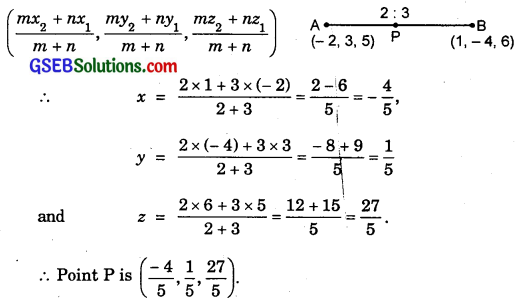∴ Point P is ($$\frac{- 4}{5}$$, $$\frac{1}{5}$$, $$\frac{27}{5}$$).

(ii) If P divides AB externally in the ratio m : n, then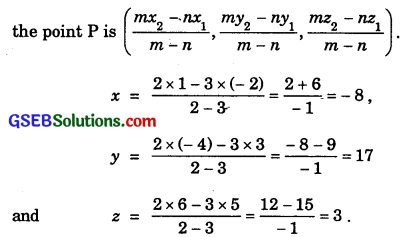∴ The point (- 8, 17, 3) divides AB externally in the ratio 2 : 3.Question 2.
Given that P(3, 2, – 4), Q(5, 4, – 6) and R(9, 8, – 10) are collinear. Find the ratijo in which Q divides PR.
Solution:
Let Q divides Pit in the ratio k : 1.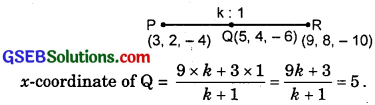or 9k + 3 = 5(k + 1) = 5k + 5 or 4k = 2
∴ k = $$\frac{1}{2}$$.
y-coordinate of Q
= $$\frac{8×k+2×1}{k+1}$$ = $$\frac{8k+2}{k+1}$$ = 4.
or 8k + 2 = 4k + 4 or 4k = 2 ⇒ k = $$\frac{1}{2}$$.
z-coordinate of Q = $$\frac{-10×k-1×4}{k+1}$$ = $$\frac{-10k-4}{k+1}$$ = – 6
or – 10k – 4 = – 6k – 6 or 4k = 2 ⇒ k = $$\frac{1}{2}$$.
This shows that the point Q lies on it and divides PR in the ratio 1 : 2.Question 3.
Find the ratio in which YZ-plane divides the line segment joining the points (- 2, 4, 7) and (3, – 5, 8).
Solution:
Let the line segment joining the points A(- 2, 4, 7) and B(3, – 5, 8) is divided by the YZ-plane at P(0, y1, z1) in the ratio k : 1.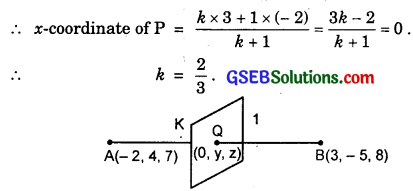i.e., YZ-plane divides AB in the ratio 2 : 3.Question 4.
Using section formula, show that the points A(2, – 3, 4), B(- 1, 2, 1) and C(0, $$\frac{1}{3}$$, 2) are collinear.
Solution:
Let the point C divides AB in the ratio k : 1.
∴ x-coordinate of C
$$\frac{-k+2}{k+1}$$ = 0 ⇒ k = 2.
y-coordinate of C
$$\frac{2k-3}{k+1}$$ = $$\frac{1}{3}$$ or 6k – 9 = k + 1 or 5k = 10 ⇒ k = 2.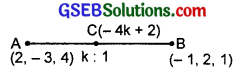z-coordinate of C
$$\frac{k+4}{k+1}$$ = 2 or k + 4 = 2k + 2 ⇒ k = 2.
This shows that C lies on AB and divides AB in the ratio 2 : 1.
Hence A, C, B are collinear.Question 5.
Find the coordinates of the points which trisect the line segment PQ formed by joining the points P(4, 2, – 6) and Q(10, – 16, 6).
Solution:
Let A(x1, y1, z1) and B(x2, y2, z2) trisect the line segment PQ.
⇒ A divides PQ in the ratio 1 : 2
∴ The coordinates of A(x1, y1, z1) are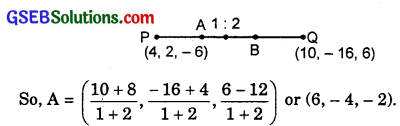Further, B divides PQ in the ratio 2 : 1.
∴ The coordinates of B(x2, y2, z2) are
($$\frac{2×10+4}{2+1}$$, $$\frac{-32+2}{2+1}$$, $$\frac{12-6}{3}$$), i.e; (8, – 10, 2).
Thus, points A(6, – 4, – 2) and B(8, – 10, 2) trisect the line segment PQ.